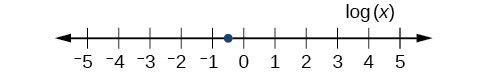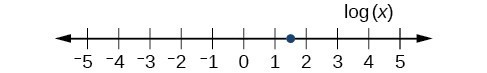## Section Exercises

1. With what kind of exponential model would half-life be associated? What role does half-life play in these models?

2. What is carbon dating? Why does it work? Give an example in which carbon dating would be useful.

3. With what kind of exponential model would doubling time be associated? What role does doubling time play in these models?

4. Define Newton’s Law of Cooling. Then name at least three real-world situations where Newton’s Law of Cooling would be applied.

5. What is an order of magnitude? Why are orders of magnitude useful? Give an example to explain.

6. The temperature of an object in degrees Fahrenheit after t minutes is represented by the equation $T\left(t\right)=68{e}^{-0.0174t}+72$. To the nearest degree, what is the temperature of the object after one and a half hours?

For the following exercises, use the logistic growth model $f\left(x\right)=\frac{150}{1+8{e}^{-2x}}$.

7. Find and interpret $f\left(0\right)$. Round to the nearest tenth.

8. Find and interpret $f\left(4\right)$. Round to the nearest tenth.

9. Find the carrying capacity.

10. Graph the model.

11. Determine whether the data from the table could best be represented as a function that is linear, exponential, or logarithmic. Then write a formula for a model that represents the data.

 x f (x) –2 0.694 –1 0.833 0 1 1 1.2 2 1.44 3 1.728 4 2.074 5 2.488

12. Rewrite $f\left(x\right)=1.68{\left(0.65\right)}^{x}$ as an exponential equation with base e to five significant digits.

For the following exercises, enter the data from each table into a graphing calculator and graph the resulting scatter plots. Determine whether the data from the table could represent a function that is linear, exponential, or logarithmic.

13.

 x f (x) 1 2 2 4.079 3 5.296 4 6.159 5 6.828 6 7.375 7 7.838 8 8.238 9 8.592 10 8.908

14.

 x f(x) 1 2.4 2 2.88 3 3.456 4 4.147 5 4.977 6 5.972 7 7.166 8 8.6 9 10.32 10 12.383

15.

 x f(x) 4 9.429 5 9.972 6 10.415 7 10.79 8 11.115 9 11.401 10 11.657 11 11.889 12 12.101 13 12.295

16.

 x f(x) 1.25 5.75 2.25 8.75 3.56 12.68 4.2 14.6 5.65 18.95 6.75 22.25 7.25 23.75 8.6 27.8 9.25 29.75 10.5 33.5

For the following exercises, use a graphing calculator and this scenario: the population of a fish farm in t years is modeled by the equation $P\left(t\right)=\frac{1000}{1+9{e}^{-0.6t}}$.

17. Graph the function.

18. What is the initial population of fish?

19. To the nearest tenth, what is the doubling time for the fish population?

20. To the nearest whole number, what will the fish population be after 2 years?

21. To the nearest tenth, how long will it take for the population to reach 900?

22. What is the carrying capacity for the fish population? Justify your answer using the graph of P.

23. A substance has a half-life of 2.045 minutes. If the initial amount of the substance was 132.8 grams, how many half-lives will have passed before the substance decays to 8.3 grams? What is the total time of decay?

24. The formula for an increasing population is given by $P\left(t\right)={P}_{0}{e}^{rt}$ where ${P}_{0}$ is the initial population and > 0. Derive a general formula for the time t it takes for the population to increase by a factor of M.

25. Recall the formula for calculating the magnitude of an earthquake, $M=\frac{2}{3}\mathrm{log}\left(\frac{S}{{S}_{0}}\right)$. Show each step for solving this equation algebraically for the seismic moment S.

26. What is the y-intercept of the logistic growth model $y=\frac{c}{1+a{e}^{-rx}}$? Show the steps for calculation. What does this point tell us about the population?

27. Prove that ${b}^{x}={e}^{x\mathrm{ln}\left(b\right)}$ for positive $b\ne 1$.

For the following exercises, use this scenario: A doctor prescribes 125 milligrams of a therapeutic drug that decays by about 30% each hour.

28. To the nearest hour, what is the half-life of the drug?

29. Write an exponential model representing the amount of the drug remaining in the patient’s system after t hours. Then use the formula to find the amount of the drug that would remain in the patient’s system after 3 hours. Round to the nearest milligram.

30. Using the model found in the previous exercise, find $f\left(10\right)$ and interpret the result. Round to the nearest hundredth.

For the following exercises, use this scenario: A tumor is injected with 0.5 grams of Iodine-125, which has a decay rate of 1.15% per day.

31. To the nearest day, how long will it take for half of the Iodine-125 to decay?

32. Write an exponential model representing the amount of Iodine-125 remaining in the tumor after t days. Then use the formula to find the amount of Iodine-125 that would remain in the tumor after 60 days. Round to the nearest tenth of a gram.

33. A scientist begins with 250 grams of a radioactive substance. After 250 minutes, the sample has decayed to 32 grams. Rounding to five significant digits, write an exponential equation representing this situation. To the nearest minute, what is the half-life of this substance?

34. The half-life of Radium-226 is 1590 years. What is the annual decay rate? Express the decimal result to four significant digits and the percentage to two significant digits.

35. The half-life of Erbium-165 is 10.4 hours. What is the hourly decay rate? Express the decimal result to four significant digits and the percentage to two significant digits.

36. A wooden artifact from an archeological dig contains 60 percent of the carbon-14 that is present in living trees. To the nearest year, about how many years old is the artifact? (The half-life of carbon-14 is 5730 years.)

37. A research student is working with a culture of bacteria that doubles in size every twenty minutes. The initial population count was 1350 bacteria. Rounding to five significant digits, write an exponential equation representing this situation. To the nearest whole number, what is the population size after 3 hours?

For the following exercises, use this scenario: A biologist recorded a count of 360 bacteria present in a culture after 5 minutes and 1000 bacteria present after 20 minutes.

38. To the nearest whole number, what was the initial population in the culture?

39. Rounding to six significant digits, write an exponential equation representing this situation. To the nearest minute, how long did it take the population to double?

For the following exercises, use this scenario: A pot of boiling soup with an internal temperature of 100º Fahrenheit was taken off the stove to cool in a 69ºF room. After fifteen minutes, the internal temperature of the soup was 95ºF.

40. Use Newton’s Law of Cooling to write a formula that models this situation.

41. To the nearest minute, how long will it take the soup to cool to 80ºF?

42. To the nearest degree, what will the temperature be after 2 and a half hours?

For the following exercises, use this scenario: A turkey is taken out of the oven with an internal temperature of 165ºF and is allowed to cool in a 75ºF room. After half an hour, the internal temperature of the turkey is 145ºF.

43. Write a formula that models this situation.

44. To the nearest degree, what will the temperature be after 50 minutes?

45. To the nearest minute, how long will it take the turkey to cool to 110ºF?

For the following exercises, find the value of the number shown on each logarithmic scale. Round all answers to the nearest thousandth.

46.47.48. Plot each set of approximate values of intensity of sounds on a logarithmic scale: Whisper: ${10}^{-10} \frac{W}{{m}^{2}}$, Vacuum: ${10}^{-4}\frac{W}{{m}^{2}}$, Jet: ${10}^{2} \frac{W}{{m}^{2}}$

49. Recall the formula for calculating the magnitude of an earthquake, $M=\frac{2}{3}\mathrm{log}\left(\frac{S}{{S}_{0}}\right)$. One earthquake has magnitude 3.9 on the MMS scale. If a second earthquake has 750 times as much energy as the first, find the magnitude of the second quake. Round to the nearest hundredth.

For the following exercises, use this scenario: The equation $N\left(t\right)=\frac{500}{1+49{e}^{-0.7t}}$ models the number of people in a town who have heard a rumor after t days.

50. How many people started the rumor?

51. To the nearest whole number, how many people will have heard the rumor after 3 days?

52. As t increases without bound, what value does N(t) approach? Interpret your answer.

For the following exercise, choose the correct answer choice.

53. A doctor and injects a patient with 13 milligrams of radioactive dye that decays exponentially. After 12 minutes, there are 4.75 milligrams of dye remaining in the patient’s system. Which is an appropriate model for this situation?

A. $f\left(t\right)=13{\left(0.0805\right)}^{t}$
B. $f\left(t\right)=13{e}^{0.9195t}$
C. $f\left(t\right)=13{e}^{\left(-0.0839t\right)}$
D. $f\left(t\right)=\frac{4.75}{1+13{e}^{-0.83925t}}$